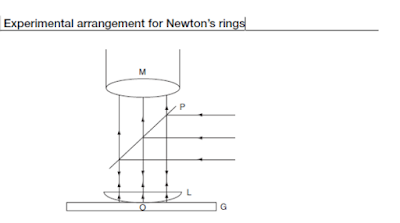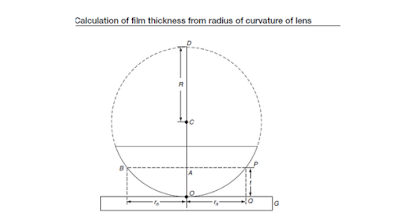Skip to main content

# Newton’s rings

The thickness of the slowly increasing air film will form between the flat glass plate and the convex surface of the flat convex lens. The thickness of the air film will be zero at the point of contact and increases symmetrically as we move radially from the point of contact. The monochromatic wavelength light "λ" is allowed to fall normally on the lens with the help of a glass plate "P", which is held at 45 ° on the incident monochromatic beam. A portion of the incident light is reflected on the convex surface of the lens and the remaining light is transmitted through the air film. Then, a portion of this transmitted light is reflected from the upper surface of the glass plate (G).Both reflected rays combine to produce an interference pattern in the form of alternating flashes and a deep concentric ring known as Newton's rings.Arrangement of experiment of Newton’s rings

Newton first demonstrated and demonstrated these rings. The rings are spherical because the air film has spherical symmetry. These rings can be viewed through the mobile microscope .Newton’s rings as seen through microscope

## Newton’s rings by reflected light theory

Let's discuss the interference conditions for bright and dark stripes and also the space between Newton's rings. To obtain the relationship between the radius of Newton's ring and the radius of curvature of the lens, consider the diagram Let the lens contact the glass plate at O and let the radius of curvature of the lens be R. Let go of a perpendicular ray. Partially reflected and partially transmitted in 'P'. The transmitted light is again reflected on the glass plate g in q. Let the thickness of the air film be PQ (= t) at P and the radius of the Newton ring at Q be rn. The reflected beam in Q undergoes a phase change different from the change or path λ / 2. The total path difference between two rays reflected in P 'and is Q' isCalculation of film thickness from radius of curvature of lens

Th e lens is a part of sphere of radius ‘R’ with center‘C ’. From the property of circle
BA×AP= OA ×AD

### Newton’s rings formula

Newton's ring formula are shown in given figure belowNewton’s rings formula

### About N-Type and P-Type Semiconductor | Materials | Example |

Here you will know about  n type and p type semiconductor in details. You can already see about bonding in semiconductor . N type and P type semiconductor is used in electronic appliances.which is the basis of working of all the electronic devices.So lets discuss in this given topic in the details. N type and P Type Semiconductor When some impurities added to the pure semiconductor then it becomes extrinsic semiconductor and this process of adding impurities is known as doping.  Now it depends on the which type of impurities it added to the semiconductor (two types of impurities can be added to semiconductor pentavalent or trivalent). n-type semiconductor : If impurity that is added to the pure semiconductor is a pentavalent ( which has five valance electron ) then it is called n-type semi conductor. p-type semiconductor : If trivalent impurity is added to the semiconductor then it becomes p-type semi conductor. ( Note-List of  n type and p type semiconductor material is given below.

### Biasing of p–n Junction | Forward and Reverse-biased |

Biasing the p-n junction means applying some external voltage to both sides of the p-n junction. Reverse-biased junction and forward-biased junction are two types of biased junction. You should have also good understanding of  N-Type and P-Type Semiconductor before moving towards this topic. Biasing of p–n Junction  Biasing of a p–n junction  means uses of some external voltage across the two sides of the p–n junction. It is divided into two groups  Forward-biased junction :  When the p-side of junction  is connected to the positive terminal of a battery and the n-side of junction is connected to the negative terminal, the p–n junction is said to be a forward-biased junction.  Reverse-biased junction  :If the positive terminal of the battery is connected to the n-side of junction and the negative terminal on the p-side, the p–n junction is said to be a reverse-biased junction. Now we will see about forward and reverse-biased junction in detail.  Related Topic    Bonding in semiconduc

### Engineers Files Explained | Types of file

Today we will learn about engineers files explained, as we know hand tools are used to remove small amounts of material, usually from small areas of the workpiece.   Do you know why hand tools are used ?Hand tools are used because of following reason: If no machine is available,  The workpiece is too large to go on a machine  The shape is too intricate or simply that it would be too expensive to set up a machine to do the work. As you know the necessity of a hand tools now we will know about a hand tool , engineer's files. Engineer’s files Files are used to perform a variety of tasks, from simple removal of sharp edges to producing complex shapes where use of the device is impractical.Files can be obtained in a variety of shapes and in lengths from 150 mm to 350 mm. Single-cut file: When a file has a single series of teeth cut across its face it is called  single-cut file ,Figure of single-cut file is shown below. double-cut file: When a file has double series of teeth cut across